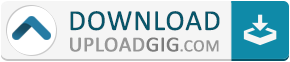#Do not remember me!

### Categories» » » Power System Analysis - Part 3: Power Flow and Short Circuit

## Power System Analysis - Part 3: Power Flow and Short CircuitMP4 | Video: h264, 1280x720 | Audio: AAC, 44.1 KHz, 2 Ch
Genre: eLearning | Language: English + .srt | Duration: 10 lectures (1h 26m) | Size: 212.9 MB

Learn about power flow analysis and short circuit analysis to further your career in electrical and power engineering
What you'll learn:
Calculate the bus admittance matrix of a power system
Short circuit analysis of balanced and unbalanced faults
Requirements
Basic knowledge of AC circuits
Prior knowledge of circuit analysis
Description
This course is the third part of a multi-part course series about one of the main areas of electrical engineering: power system analysis. Power system analysis is the core of power engineering and its understanding is therefore essential for a career in this field. In this third course of the multi-part course series, you will learn about power flow (load flow) analysis and short circuit analysis and their use in power systems. The course is divided into the following sections:
1. Power Flow (Load Flow) Analysis: in section 2, we will introduce the concept of power flow. Also referred to as load flow, power flow is the analysis of how apparent, real, and reactive power flows between parts of a power system, from generation to the loads. Two different methods will be covered, which are the most widely used methods in power system analysis: the Gauss-Seidel method and the Newton-Raphson method. Several examples will be solved to help explain how these methods are used for power flow analysis.
2. Short Circuit Analysis of Balanced Faults: in section 3, we will introduce short circuits. Also referred to as faults, short circuits are undesired occurrences in power systems when conductors are shorted between each other, to ground, or a combination of these. This is the basis for the field of protection and control which is widely important for the safe and reliable operation of power systems. To introduce how short circuits (faults) affect power systems, we will begin by discussing balanced (i.e., three-phase) short circuits. We will also introduce the concept of the short circuit capacity and the bus impedance matrix.
3. Short Circuit Analysis of Unbalanced Faults: in section 4, we will continue discussing short circuits (faults), but will discuss the more complex analysis of unbalanced faults (e.g., single-line-to-ground, line-to-line, and line-to-line-to-ground faults). To do this, we will introduce the technique of symmetrical components, which allows us to analyze unbalanced power systems more easily.
In each section, several examples are solved to illustrate how to analyze real-world power systems.
By learning about power flow analysis and short circuit analysis and how they are used in power systems, you will be able to continue your study of power system analysis for a career in power engineering and electrical engineering.
Remember that Udemy offers a 30-day money-back guarantee. I am also always available for questions while you go through the course to ensure everything is clear.
See you in the course!
Who this course is for
Anybody with an interest in learning about power systems and power engineeringName:* E-Mail: Security Code: *